Question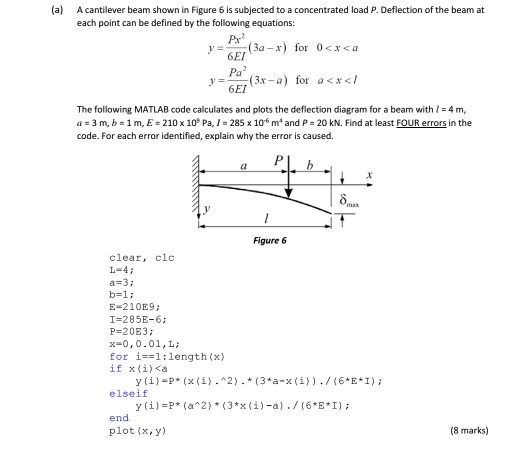Here is the given code:

clear,clc;

L=4;

a=3;

b=1;

E=210E9;

I=285E-6;

P=20E3;

X=0,0.01,L;

for i==1:length(X);

if X(i)<a

Y(i)=p*(x(i).^2).*(3*a-X(i))./(6*E*I);

elseif

Y(i)=p*(a^2)*(3*X(i)-a)./(6*E*I);

end

plot(X,Y)

These are the errors in the code:

1)X=0,0.1,L

But it gives error.

Because X has to be given values in the range 0 to L with increment 0.1.but it cann't.so corret syntax for it is   X=0:0.1:L.

2) for i==1:length(X)

But it gives error.

Because i==1 is used for equlity condition not for the assignmet to the range.

so correct syntax is for i=1:length(X)

3)

elseif

Y(i)=p*(a^2)*(3*X(i)-a)./(6*E*I);

But it gives error.

Because, elseif always require a condition to execute the statement.so here we se the correct control statement is else.

else

Y(i)=p*(a^2)*(3*X(i)-a)./(6*E*I);

4)

for i==1:length(X)

if X(i)<a

Y(i)=p*(x(i).^2).*(3*a-X(i))./(6*E*I);

else

Y(i)=p*(a^2)*(3*X(i)-a)./(6*E*I);

end

But it gives error.

Because we have end the for loop.but here we cant end the for loop.so we end after the for loop.

correct syntax is :

for i=1:length(X)

if X(i)<a

Y=p*(x(i)^2).*(3*a-X(i))./(6*E*I);

else

Y=p*(a^2)*(3*X(i)-a)./(6*E*I);

end

end

5) Y(i)=p*(x(i)^2).*(3*a-X(i))./(6*E*I);

here Y(i) is not required.so we use only Y.correct syntax is Y=p*(x(i)^2).*(3*a-X(i))./(6*E*I);

#### Earn Coins

Coins can be redeemed for fabulous gifts.

Similar Homework Help Questions
• ### 1. The cantilever beam shown in the following figure is subjected to a load P or...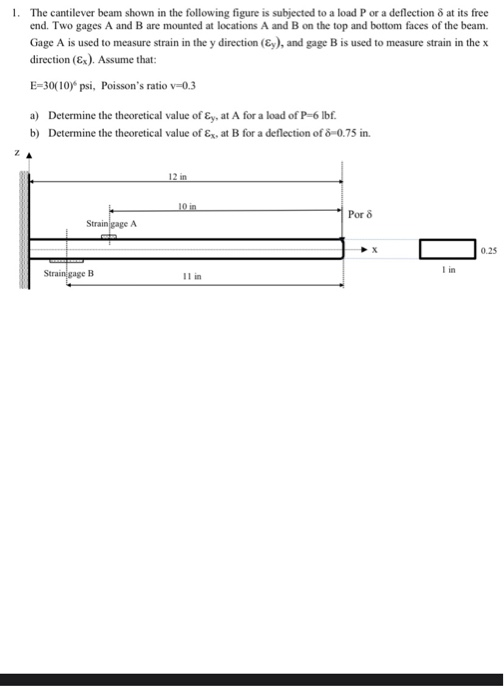1. The cantilever beam shown in the following figure is subjected to a load P or a deflection o at its free end. Two gages A and B are mounted at locations A and B on the top and bottom faces of the beam. Gage A is used to measure strain in the y direction (Ey), and gage B is used to measure strain in the x direction (Ex). Assume that: E=30(10) psi, Poisson's ratio v=0.3 a) Determine the theoretical...

• ### The cantilever beam shown is subjected to a concentrated load of P = 46200 lb. The...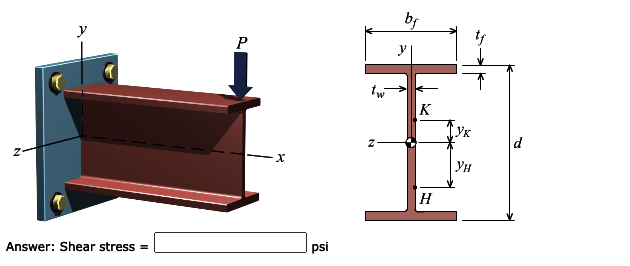The cantilever beam shown is subjected to a concentrated load of P = 46200 lb. The cross-sectional dimensions and the moment of inertia of the W16x40 wide-flange shape are: d = 16.0 in. tw = 0.305 in. bf = 7.00 in. tf = 0.505 in. Iz = 518 in.4 Compute the value of the shear stress at point K, located at yK = 2.4 in. above the centroidal axis. by P y T tw K Lyk Z d x Ун...

• ### The cantilever beam is subjected to a concentrated load of P = 29 kips. The cross-sectional dimen...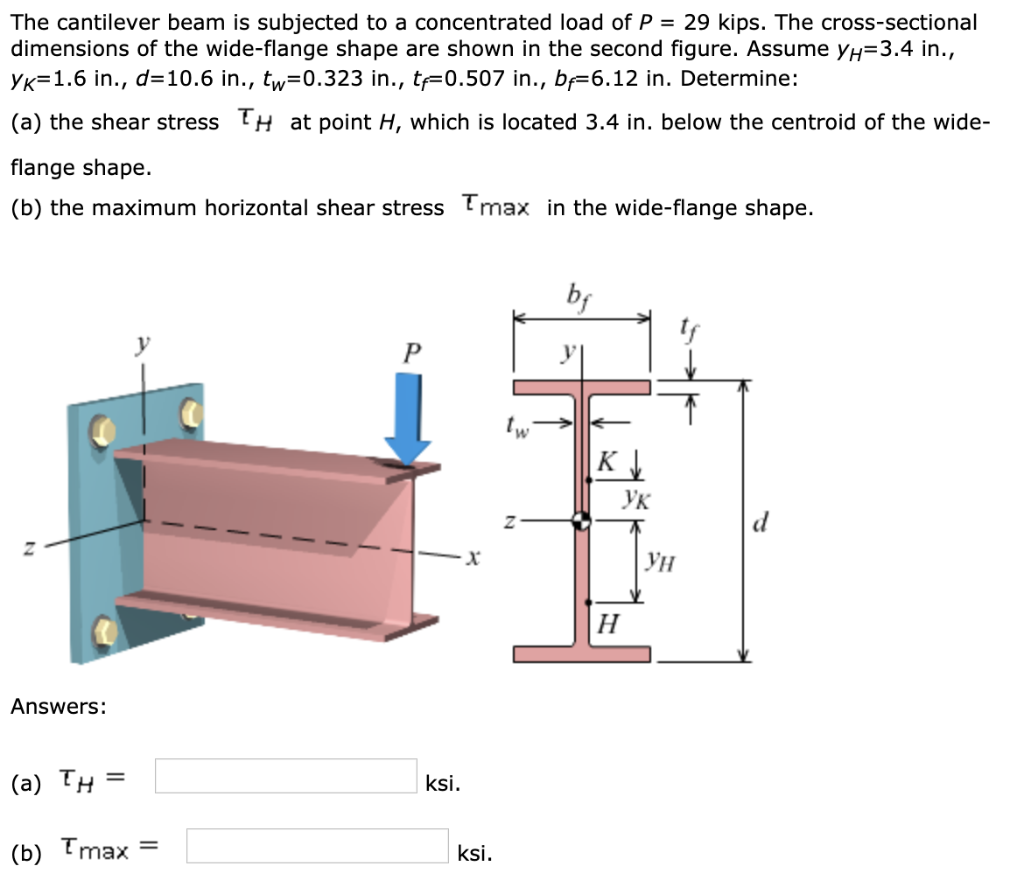The cantilever beam is subjected to a concentrated load of P = 29 kips. The cross-sectional dimensions of the wide-flange shape are shown in the second figure. Assume yH=3.4 in., yK=1.6 in., d=10.6 in., tw=0.323 in., tf=0.507 in., bf=6.12 in. Determine: The cantilever beam is subjected to a concentrated load of P 29 kips. The cross-sectional dimensions of the wide-flange shape are shown in the second figure. Assume y,-3.4 in., Ук_ 1.6 in., d-10.6 in., t,-0.323 in., tf-0.507 in., bf-6.12...

• ### A cantilever beam AB is subjected to a triangle loading with concentrated moment (see figure). The...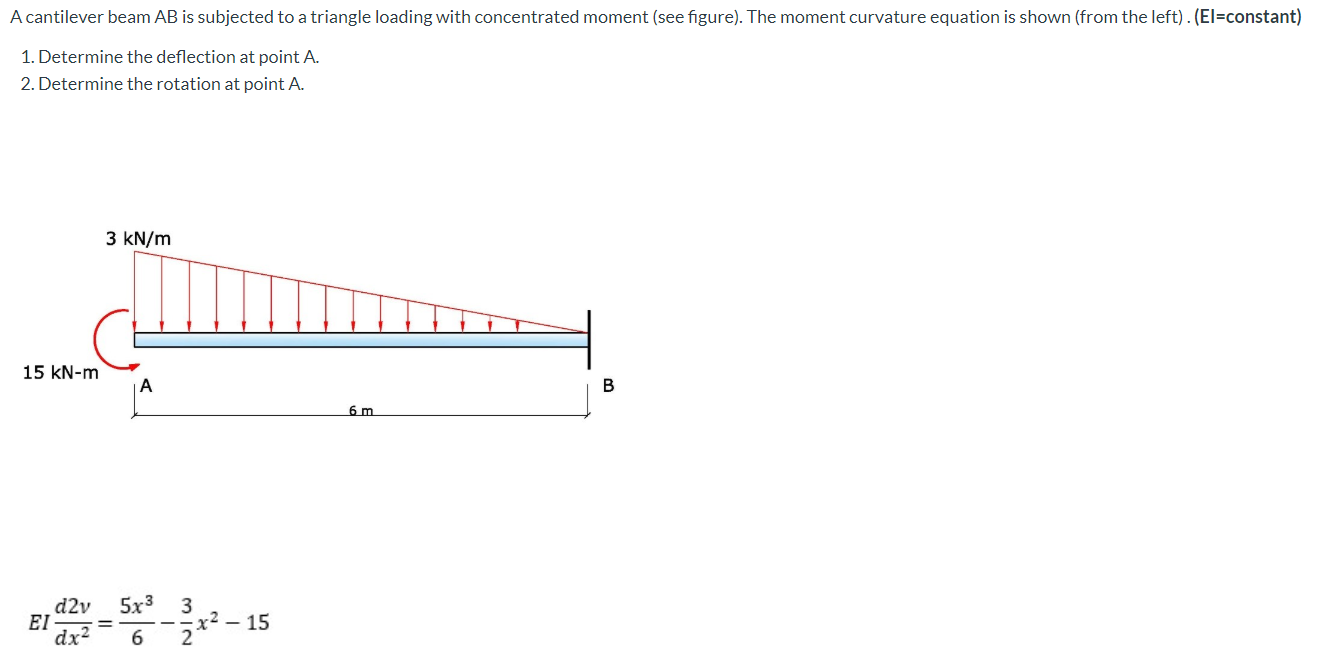A cantilever beam AB is subjected to a triangle loading with concentrated moment (see figure). The moment curvature equation is shown (from the left). (El=constant) 1. Determine the deflection at point A. 2. Determine the rotation at point A. 3 kN/m 15 kN-m A B 6 m d2v ΕΙ 5x3 3 x2 – 15 2 dx2 6

• ### The cantilever beam is subjected to a concentrated load of P = 52 kips. The cross-sectional...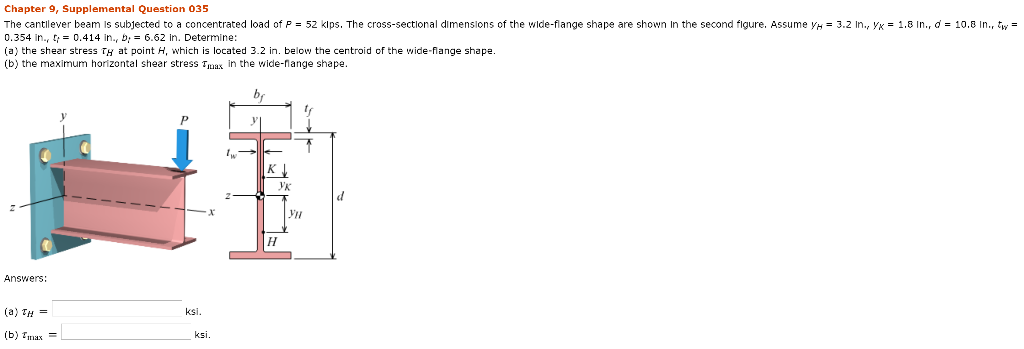The cantilever beam is subjected to a concentrated load of P = 52 kips. The cross-sectional dimensions of the wide-flange shape are shown in the second figure. Assume yH = 3.2 in., yK = 1.8 in., d = 10.8 in., tw = 0.354 in., tf = 0.414 in., bf = 6.62 in. Determine: (a) the shear stress τH at point H, which is located 3.2 in. below the centroid of the wide-flange shape. (b) the maximum horizontal shear stress τmax...

• ### The cantilever beam shown in the figure is subjected to a concentrated load at point B....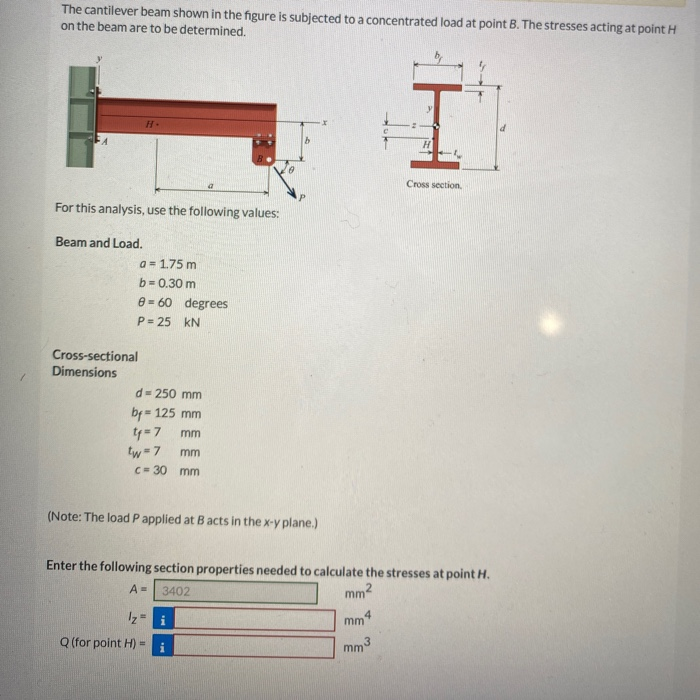The cantilever beam shown in the figure is subjected to a concentrated load at point B. The stresses acting at point H on the beam are to be determined. H Cross section For this analysis, use the following values: Beam and Load. a = 1.75 m b=0.30 m @= 60 degrees P = 25 KN Cross-sectional Dimensions d=250 mm bp = 125 mm ty=7 mm tw = 7 mm C= 30 mm (Note: The load P applied at Bacts in...

• ### The cantilever beam shown is subjected to a concentrated load of P = 34500 lb. The...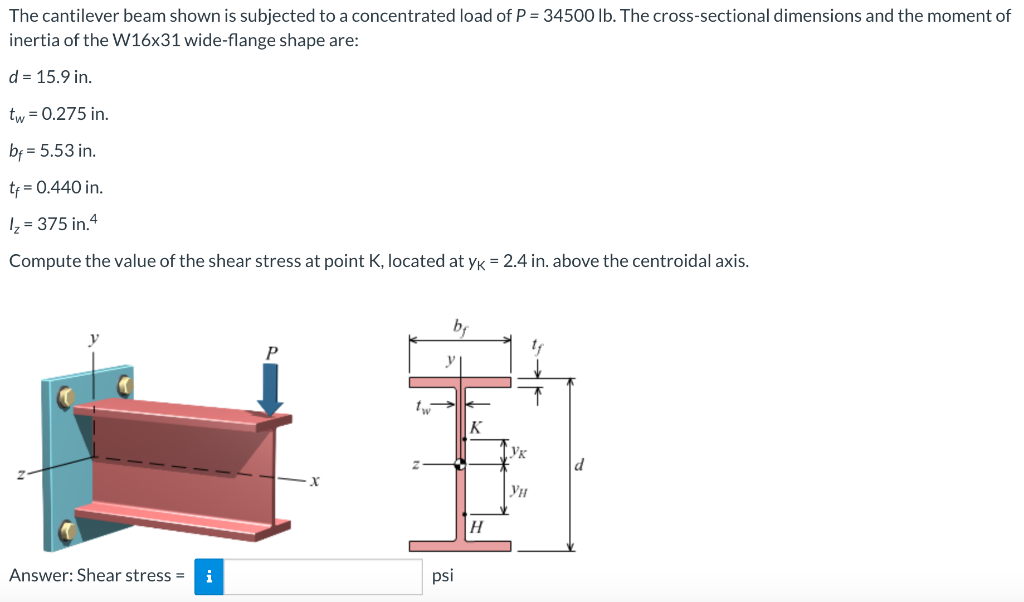The cantilever beam shown is subjected to a concentrated load of P = 34500 lb. The cross-sectional dimensions and the moment of inertia of the W16x31 wide-flange shape are: d = 15.9 in. tw = 0.275 in. be= 5.53 in. tp = 0.440 in. 12 = 375 in 4 Compute the value of the shear stress at point K, located at yk = 2.4 in. above the centroidal axis. bi 11 y 1 K Ук | Ун H Answer: Shear...

• ### The cantilever beam shown in the figure is subjected to a concentrated load at point B....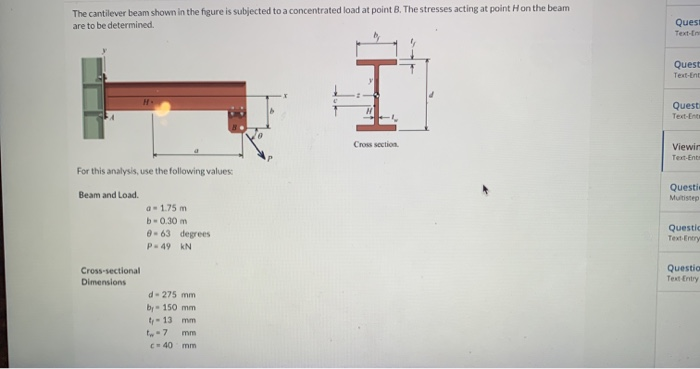The cantilever beam shown in the figure is subjected to a concentrated load at point B. The stresses acting at point Hon the beam are to be determined Ques! Text- Quest Text Ent T Quest Text End Cross section Viewir Text-Ent For this analysis, use the following values Beam and Load. Questi Muitstep a. 1.75 m b-0.30 m 0.63 degrees P.49 KN Questid Text Entry Questio Text Entry Cross-sectional Dimensions d - 275 mm by - 150 mm - 13...

• ### The cantilever beam is subjected to a concentrated load of P = 35 kips. The cross-sectional...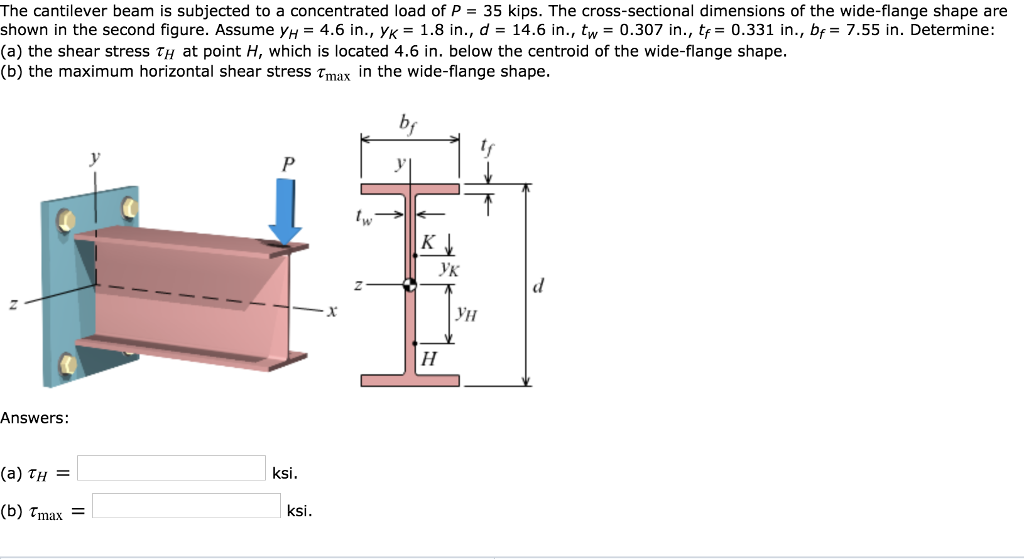The cantilever beam is subjected to a concentrated load of P = 35 kips. The cross-sectional dimensions of the wide-flange shape are shown in the second figure. Assume yH = 4.6 in., ?? 1.8 in, d = 14.6 in., tw= 0.307 in., tf= 0.331 in., br= 7.55 in. Determine: (a) the shear stress tH at point H, which is located 4.6 in. below the centroid of the wide-flange shape. (b) the maximum horizontal shear stress Tmax in the wide-flange shape...

• ### A cantilever beam of length L and constant El carries a concentrated load P at a...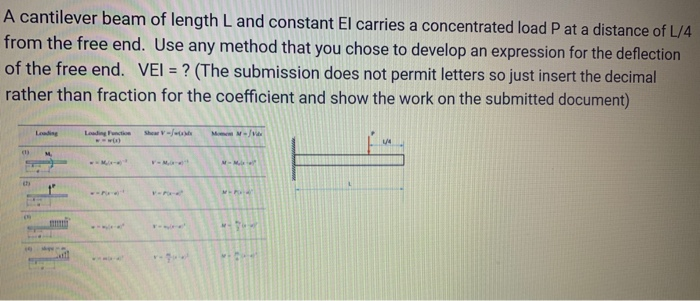A cantilever beam of length L and constant El carries a concentrated load P at a distance of L/4 from the free end. Use any method that you chose to develop an expression for the deflection of the free end. VEI = ? (The submission does not permit letters so just insert the decimal rather than fraction for the coefficient and show the work on the submitted document) Loading Functie Men - M